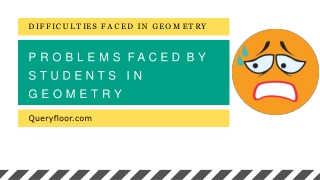DownloadDownload Presentationproblems faced by students in geometry

# problems faced by students in geometry

Download Presentation## problems faced by students in geometry

- - - - - - - - - - - - - - - - - - - - - - - - - - - E N D - - - - - - - - - - - - - - - - - - - - - - - - - - -
##### Presentation Transcript

1. D I F F I C U L T IES F AC ED IN G E O M E T RY PROBLEMSFACEDBY STUDENTS IN GEOMETRY Queryfloor.com

2. LOTSOFFORMULASANDTHEOREMS Students get lots of problems in highschool geometry. There are lots of formulas, theorems, and steps in differentproblems. The formulas of one problem may merge with another and students do not get an idea of the application. It becomes toughfor the students to prove theformulas. COMPLICATED CONCEPTS Concepts Queryfloor.com

3. INTERCONNECTION There are many formulas in geometry to be remembered. Students have to remember them all during the exams and also to learn new geometry lessons. Every problem inthe subject includes formulas which are interconnected with each other. So, if you have one geometric problem, you need to apply many formulas to arrive at the solution. NUMEROUS FORMULAS Queryfloor.com

4. Students do not get goodmarks ALLTHEGEOMETRYLESSONSAREINTERRELATED TO EACH OTHER. ONE WRONG STEP IN THE GEOMETRIC PROBLEM WILL GIVE THE WRONG ANSWERATTHEEND.EVERYSTEPISTHEPROBLEM HASMARKSANDSTUDENTSFAILTOGETGOOD MARKSINTHEEXAMS.THISISTHEREASONWHY MANYSTUDENTSFAILINGEOMETRYEXAMS. Queryfloor.com

5. The real scenario is that most of the students findgeometrymathboringandso,theydonot practice the sums. As a result, they do not understand where to apply the theorems and formulas. LACK OF PRACTICAL KNOWLEDGE To score well in geometry, it is necessary to practice the geometry problems every day. By practicing more geometric problems,you can know the application of various geometry theorems and formulas in the sums. QUERYFLOOR.COM

6. L ACK O F MATH BASE Tobegoodatgeometry,itisimportanttofirst learn math basics. Lack of basic geometry lessonsisthemainreasonwhystudentsfind this subjectdifficult. Queryfloor.com

7. AFFORDABLE TEACHING Extracoaching classes Geometry needs extra coaching classes by well-educated teachers. This is quite an expensivethingwhichmanystudentscannot afford. This will further bring them lots of problems in theexams. Queryfloor.com

8. Uninteresting Geometrysubject Students do not know where to apply the formulas in the geometry problems. Most of studentsforgetmathematical formulas which make the subject reallyboring. Queryfloor.com

9. Lack of personal attention Teachers should give personal attention to the students for teaching various geometry problems. But today, the schools have to complete a vast syllabus in the limited time. So, the students do not get enough attention due to which they find difficult to solve geometry sums in exams also. QUERYFLOOR.COM

10. THANKYOU QUERYFLOOR.COM